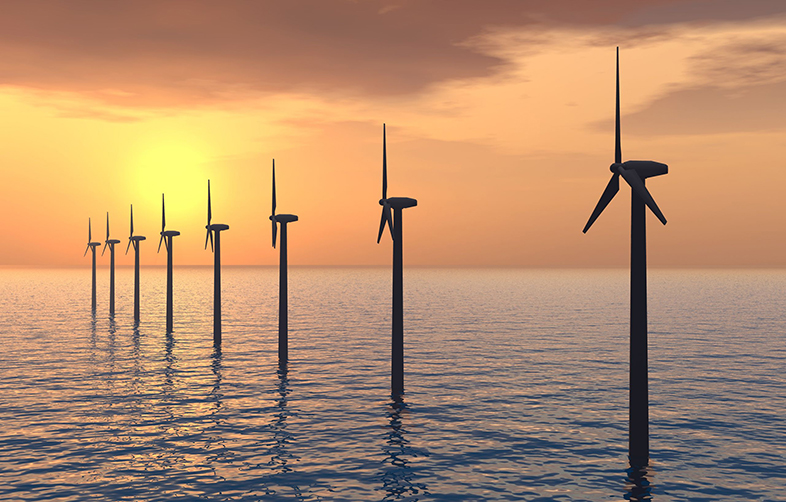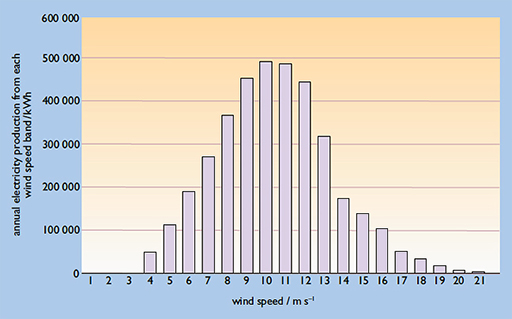Science, Maths & Technology

### Become an OU studentCan renewable energy sources power the world?

Start this free course now. Just create an account and sign in. Enrol and complete the course for a free statement of participation or digital badge if available.

# 5.1 Calculating wind energy distribution

For each incremental wind speed between the cut-in wind speed and the shut-down wind speed the energy produced can be obtained by using this equation:

This data can then be used to plot a wind speed – annual output energy distribution graph, such as that shown in Figure 12 .Figure 12 Example of a wind speed – annual energy output distribution curve, showing annual electricity production at each wind speed

The total energy produced in a year is then calculated by summing the all the energies produced at each wind speed within the operating range of the turbine.

The wind speed distribution at a site is provided by measuring equipment that records the number of hours for which the wind speed lies within each one metre per second (1 m s−1) wide speed ‘band’, e.g:

• 0–1 m s−1
• 1–2 m s−1
• 2–3 m s−1, etc.

The longer the period over which measurements are taken, the more accurate is the estimate of the wind speed frequency distribution.

## Activity 1 Factors on total energy generated

What other factors could affect the total energy generated?